Click to Chat

1800-1023-196

+91-120-4616500

CART 0

• 0

MY CART (5)

Use Coupon: CART20 and get 20% off on all online Study Material

ITEM
DETAILS
MRP
DISCOUNT
FINAL PRICE
Total Price: Rs.

There are no items in this cart.
Continue Shopping• Complete JEE Main/Advanced Course and Test Series
• OFFERED PRICE: Rs. 15,900
• View Details

```Chapter 14: Compound Interest Exercise – 14.1

Question: 1

Find the compound interest when principal = Rs 3000, rate = 5% per annum and time = 2 years.

Solution: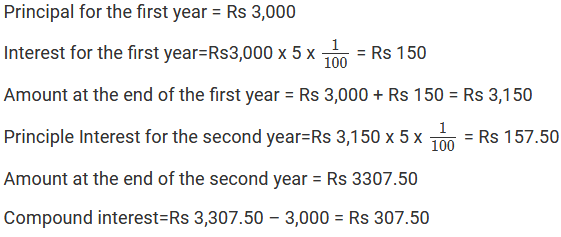Question: 2

What will be the compound interest on Rs 4000 in two years when the rate of interest is 5% per annum?

Solution: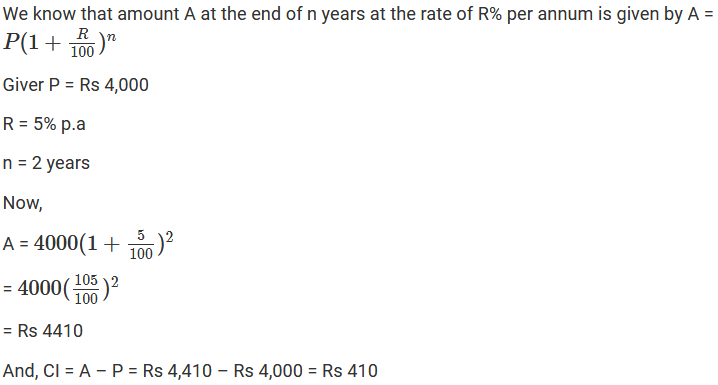Question: 3

Rohit deposited Rs 8000 with a finance company for 3 years at an interest of 15% per annum. What is the compound interest that Rohit gets after 3 years?

Solution: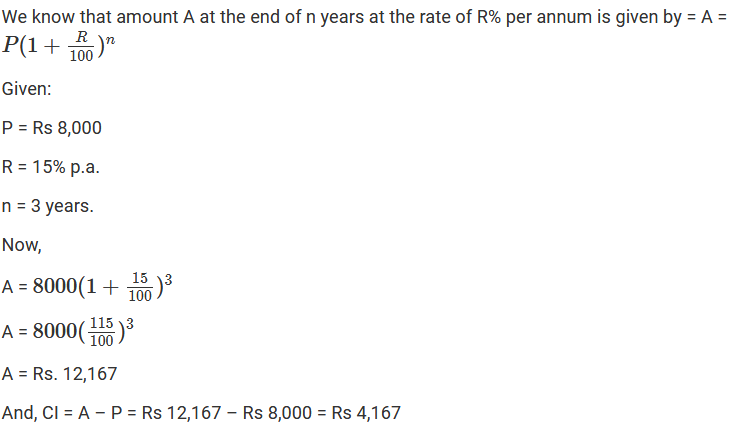Question: 4

Find the compound interest on Rs 1000 at the rate of 8% per annum for 1(1/2) years when interest is compounded half-yearly.

Solution: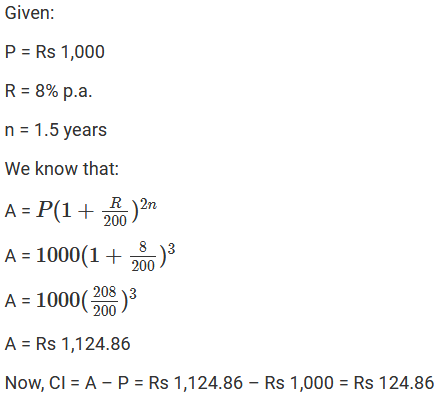Question: 5

Find the compound interest on Rs 160000 for one year at the rate of 20% per annum, if the interest is compounded quarterly.

Solution:Question: 6

Swati took a loan of Rs 16000 against her insurance policy at the rate of 12(1/2)% per annum. Calculate the total compound interest payable by Swati after 3 year.

Solution:Question: 7

Roma borrowed Rs 64000 from a bank for 1(1/2) years at the rate of 10% per annum. Compute the total compound interest payable by Roma after 1(1/2) years, if the interest is compounded half-yearly.

Solution: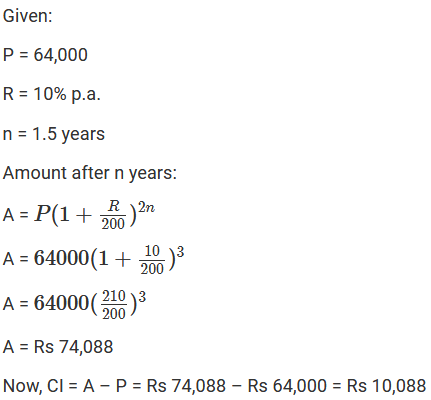Question: 8

Mewa Lal borrowed Rs 20000 from his friend Rooplal at 18% per annum simple interest. He lent it to Rampal at the same rate but compounded annually. Find his gain after 2 years.

Solution:Question: 9

Find the compound interest on Rs 8000 for 9 months at 20% per annum compounded quarterly.

Solution:Question: 10

Find the compound interest at the rate of 10% per annum for two years on that principle which in two years at the rate of 10% per annum gives Rs 200 as simple interest.

Solution: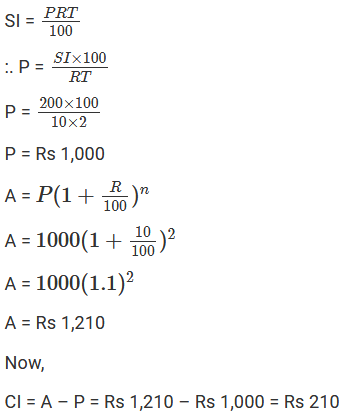Question: 11

Find the compound interest on Rs 64000 for 1 year at the rate of 10% per compounded quarterly.

Solution: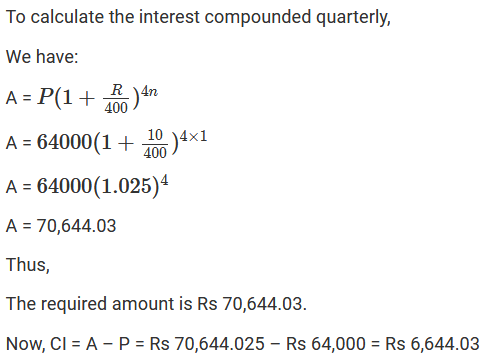Question: 12

Ramesh deposited Rs 7500 in a bank which pays him 12% interest per annum compounded quarterly. What is the amount which he receives after 9 months?

Solution: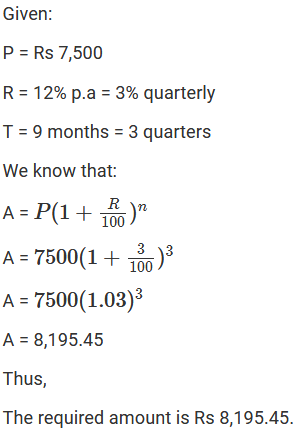Question: 13

Anil borrowed a sum of Rs 9600 to install a hand pump in his dairy. If the rate of 1 interest is 5(1/2)% per annum compounded annually, determine the compound interest which Anil will have to pay after 3 years.

Solution: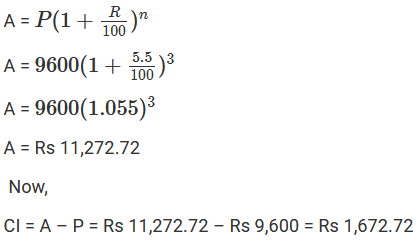Question: 14

Surabhi borrowed a sum of Rs 12000 from a finance company to purchase a refrigerator. If the rate of interest is 5% per annum compounded annually, calculate the compound interest that Surabhi has to pay to the company after 3 years.

Solution: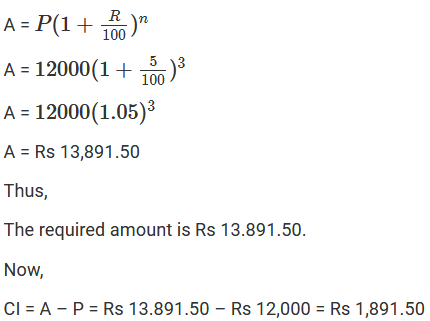Question: 15

Daljit received a sum of Rs. 40000 as a loan from a finance company. If the rate of interest is 7% per annum corn compounded annually, calculate the compound interest that Daljit pays after 2 years.

Solution: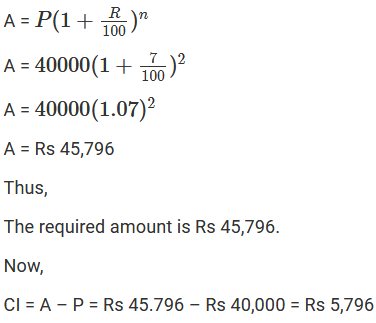```### Course Features

• 728 Video Lectures
• Revision Notes
• Previous Year Papers
• Mind Map
• Study Planner
• NCERT Solutions
• Discussion Forum
• Test paper with Video Solution